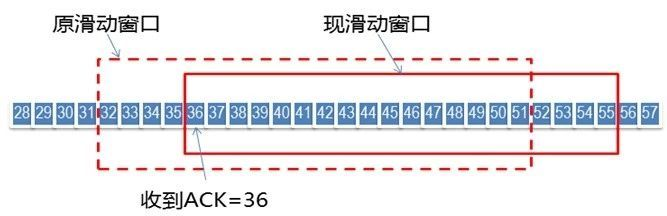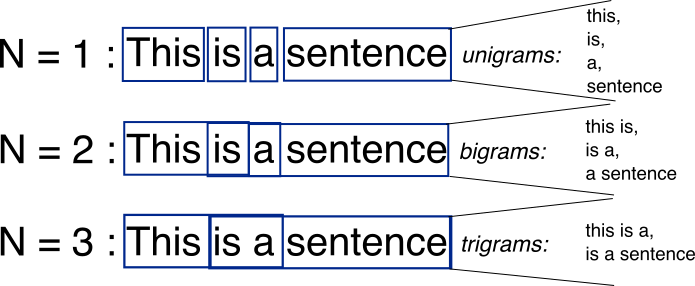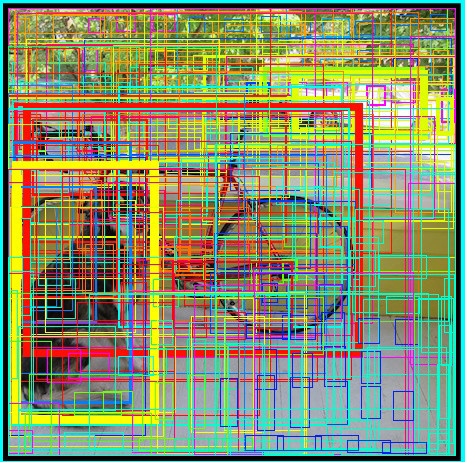﻿ 字节跳动-挑战字符串 - Nemo

# 字节跳动-挑战字符串[TOC]

## 1.无重复字符的最长子串

输入: &quot;abcabcbb&quot;

输入: &quot;bbbbb&quot;

输入: &quot;pwwkew&quot;

请注意，你的答案必须是 子串 的长度，&quot;pwke&quot; 是一个子序列，不是子串。

### 解答

“滑动窗口”这个概念在计算机算法中非常常见。该算法可以把嵌套的循环转化为单循环从而降低时间复杂度。它在很多不同的领域都有应用：

1.TCP协议的滑动窗口进行流量控制2.NLP（自然语言处理）中的 N-gram3.图像处理中的物体识别class Solution(object):
def lengthOfLongestSubstring(self, s):
&quot;&quot;&quot;
:type s: str
:rtype: int
&quot;&quot;&quot;
maxlen = 0
memo = dict()
begin, end = 0, 0
n = len(s)
while end &lt; n:
last = memo.get(s[end])
memo[s[end]] = end
if last is not None:
maxlen = max(maxlen, end-begin)
begin = max(begin, last + 1)
end += 1
maxlen = max(maxlen, end-begin)
return maxlen

def lengthOfLongestSubstring(self, s):
&quot;&quot;&quot;
:type s: str
:rtype: int
&quot;&quot;&quot;
ans = 0
start = 0
end = 0
StrSet = set()
while start &lt; len(s) and end &lt; len(s):
StrLen = len(StrSet)
if not StrLen == len(StrSet):
end += 1
ans = max(ans, end - start)
else:
StrSet.remove(s[start])
start += 1
return ans

## 2.最长公共前缀

输入: [&quot;flower&quot;,&quot;flow&quot;,&quot;flight&quot;]

输入: [&quot;dog&quot;,&quot;racecar&quot;,&quot;car&quot;]

### 解答

class Solution(object):
def longestCommonPrefix(self, strs):
&quot;&quot;&quot; :type strs: List[str] :rtype: str &quot;&quot;&quot;
res =&quot;&quot; #创建一个空字符串
if len(strs)==0:
return res

temp = strs
for str in strs:
if len(temp) &gt; len(str):
temp = str #找出最短的字符串作为模版
for i in range(0,len(temp)):
for j in range(0,len(strs)):
if strs[j][i]!=temp[i]:
return res;
res = res + temp[i]
return res;

Python 特性，取每一个单词的同一位置的字母，看是否相同。

class Solution:
def longestCommonPrefix(self, strs):
"""
:type strs: List[str]
:rtype: str
"""
res = ""
for tmp in zip(*strs):
tmp_set = set(tmp)
if len(tmp_set) == 1:
res += tmp
else:
break
return res

思路二：
PythonJava
class Solution:
def longestCommonPrefix(self, s: List[str]) -> str:
if not s:
return ""
res = s
i = 1
while i < len(s):
while s[i].find(res) != 0:
res = res[0:len(res)-1]
i += 1
return res

思路三：
PythonJava
class Solution:
def longestCommonPrefix(self, s: List[str]) -> str:
if not s:
return ""
s.sort()
n = len(s)
a = s
b = s[n-1]
res = ""
for i in range(len(a)):
if i < len(b) and a[i] == b[i]:
res += a[i]
else:
break
return res


class Solution:
def longestCommonPrefix(self, strs: List[str]) -> str:
r = [len(set(c)) == 1 for c in zip(*strs)] + 
return strs[:r.index(0)] if strs else ''


【第一行】每次都取各个字符串的同一列字符，放进 set，set 中不会储存重复元素，所以长度为1代表各个字符都是相同的，此时 == 会让它变成 True
【第二行】index 搜索第一个 0 的位置，0 与 False 在值上是等价的，相当于搜索第一个 False 的位置也就是公共前缀的长度

zip(*str) 将 str 中所有字符串并列到迭代器中，逐次并列返回 str 中所有字符串的第 1、2、3、…… 个字符

## 3.字符串的排列

输入: s1 = &quot;ab&quot; s2 = &quot;eidbaooo&quot;



输入: s1= &quot;ab&quot; s2 = &quot;eidboaoo&quot;



1. 输入的字符串只包含小写字母
2. 两个字符串的长度都在 [1, 10,000] 之间

### 解答

from collections import Counter
class Solution:
def checkInclusion(self, s1: str, s2: str) -&gt; bool:
start = 0
end = 0
need_win = Counter(s1)
lookup = Counter()
count = 0
while end &lt; len(s2):
if s2[end] not in need_win.keys():
lookup.clear()
count = 0
start = end + 1
else:
lookup[s2[end]] += 1
count += 1
end = end + 1
while count == len(s1):
while all(map(lambda x:lookup[x] &gt;= need_win[x], need_win.keys())):
if end - start == len(s1):
return True
break
lookup[s2[start]] -= 1
start = start + 1
count -= 1
return False


## 4.字符串相乘

输入: num1 = &quot;2&quot;, num2 = &quot;3&quot;



输入: num1 = &quot;123&quot;, num2 = &quot;456&quot;



1. num1num2 的长度小于110。
2. num1num2 只包含数字 0-9
3. num1num2 均不以零开头，除非是数字 0 本身。
4. 不能使用任何标准库的大数类型（比如 BigInteger）直接将输入转换为整数来处理

### 解答Python

class Solution(object):
def multiply(self, num1, num2):
"""
:type num1: str
:type num2: str
:rtype: str
"""
if num1 == "0" or num2 == "0": #处理特殊情况
return "0"

l1, l2 = len(num1), len(num2)
if l1 < l2:
num1, num2 = num2, num1 #保障num1始终比num2长， 个人习惯
l1, l2 = l2, l1

num2 = num2[::-1] #注意要倒过来乘，方便进位
res = "0"
for i, digit in enumerate(num2):
tmp = self.StringMultiplyDigit(num1, int(digit)) + "0" * i #计算num1和num2的当前位的乘积
res = self.StringPlusString(res, tmp) #计算res和tmp的和

return res


Python

def StringMultiplyDigit(self,string, n):
#这个函数的功能是：计算一个字符串和一个整数的乘积，返回字符串
#举例：输入为 "123", 3， 返回"369"
s = string[::-1]
res = []
for i, char in enumerate(s):
num = int(char)
res.append(num * n)
res = self.CarrySolver(res)
res = res[::-1]
return "".join(str(x) for x in res)


Python

def CarrySolver(self, nums):
#这个函数的功能是：将输入的数组中的每一位处理好进位
#举例：输入[15, 27, 12], 返回[5, 8, 4, 1]
i = 0
while i < len(nums):
if nums[i] >= 10:
carrier = nums[i] // 10
if i == len(nums) - 1:
nums.append(carrier)
else:
nums[i + 1] += carrier
nums[i] %= 10
i += 1


PS：第415题 就是要写这个函数

Python

def StringPlusString(self, s1, s2):
#这个函数的功能是：计算两个字符串的和。
#举例：输入为“123”， “456”, 返回为"579"
#PS：LeetCode415题就是要写这个函数
l1, l2 = len(s1), len(s2)
if l1 < l2:
s1, s2 = s2, s1
l1, l2 = l2, l1
s1 = [int(x) for x in s1]
s2 = [int(x) for x in s2]
s1, s2 = s1[::-1], s2[::-1]
for i, digit in enumerate(s2):
s1[i] += s2[i]

s1 = self.CarrySolver(s1)
s1 = s1[::-1]
return "".join(str(x) for x in s1)


Python

class Solution(object):
def multiply(self, num1, num2):
"""
:type num1: str
:type num2: str
:rtype: str
"""
if num1 == "0" or num2 == "0": #处理特殊情况
return "0"

l1, l2 = len(num1), len(num2)
if l1 < l2:
num1, num2 = num2, num1 #保障num1始终比num2大
l1, l2 = l2, l1

num2 = num2[::-1]
res = "0"
for i, digit in enumerate(num2):
tmp = self.StringMultiplyDigit(num1, int(digit)) + "0" * i #计算num1和num2的当前位的乘积
res = self.StringPlusString(res, tmp) #计算res和tmp的和

return res

def StringMultiplyDigit(self,string, n):
#这个函数的功能是：计算一个字符串和一个整数的乘积，返回字符串
#举例：输入为 "123", 3， 返回"369"
s = string[::-1]
res = []
for i, char in enumerate(s):
num = int(char)
res.append(num * n)
res = self.CarrySolver(res)
res = res[::-1]
return "".join(str(x) for x in res)

def CarrySolver(self, nums):
#这个函数的功能是：将输入的数组中的每一位处理好进位
#举例：输入[15, 27, 12], 返回[5, 8, 4, 1]
i = 0
while i < len(nums):
if nums[i] >= 10:
carrier = nums[i] // 10
if i == len(nums) - 1:
nums.append(carrier)
else:
nums[i + 1] += carrier
nums[i] %= 10
i += 1

return nums

def StringPlusString(self, s1, s2):
#这个函数的功能是：计算两个字符串的和。
#举例：输入为“123”， “456”, 返回为"579"
#PS：LeetCode415题就是要写这个函数
l1, l2 = len(s1), len(s2)
if l1 < l2:
s1, s2 = s2, s1
l1, l2 = l2, l1
s1 = [int(x) for x in s1]
s2 = [int(x) for x in s2]
s1, s2 = s1[::-1], s2[::-1]
for i, digit in enumerate(s2):
s1[i] += s2[i]

s1 = self.CarrySolver(s1)
s1 = s1[::-1]
return "".join(str(x) for x in s1)


class Solution:
def str2int(self,s):
return ord(s)-ord(&#039;0&#039;)

def multiply(self, num1: str, num2: str) -&gt; str:
a = num1[::-1]
b = num2[::-1]
result = 0

for i,x in enumerate(a):
temp_result = 0
for j,y in enumerate(b):
temp_result += self.str2int(x) * self.str2int(y) * 10**j
result += temp_result * 10**i
return str(result)


## 5.翻转字符串里的单词

输入: &quot;the sky is blue&quot;



输入: &quot;  hello world!  &quot;



输入: &quot;a good   example&quot;



• 无空格字符构成一个单词。
• 输入字符串可以在前面或者后面包含多余的空格，但是反转后的字符不能包括。
• 如果两个单词间有多余的空格，将反转后单词间的空格减少到只含一个。

### 解答

• 先处理字符串，将首尾空格都删除；
1. 倒序遍历字符串，当第一次遇到空格时，添加s[i + 1: j]（即添加一个完整单词）；
2. 然后，将直至下一个单词中间的空格跳过，并记录下一个单词尾部j
3. 继续遍历，直至下一次遇到第一个空格，回到1.步骤；
• 由于首部没有空格，因此最后需要将第一个单词加入，再return。
• python可一行实现。
class Solution:
def reverseWords(self, s: str) -&gt; str:
s = s.strip()
res = &#039;&#039;
i ,j = len(s)-1,len(s)
while i &gt; 0:
if s[i] == &#039; &#039;:
res += s[i+1:j]+&#039; &#039;
while s[i] == &#039; &#039;:
i -= 1
j = i+1
i -= 1
return res+s[:j]


Python strip() 方法用于移除字符串头尾指定的字符（默认为空格或换行符）或字符序列。

## 6.简化路径

输入：&quot;/home/&quot;



输入：&quot;/../&quot;



输入：&quot;/home//foo/&quot;



输入：&quot;/a/./b/../../c/&quot;



输入：&quot;/a/../../b/../c//.//&quot;



输入：&quot;/a//b////c/d//././/..&quot;



### 解答

class Solution:
def simplifyPath(self, path: str) -&gt; str:
stack = []
path = path.split(&#039;/&#039;)

for item in path:
if item == &#039;..&#039;:
if stack:
stack.pop()
elif item and item != &#039;.&#039;:
stack.append(item)
return &#039;/&#039;+&#039;/&#039;.join(stack)


## 7.复原IP地址

输入: &quot;25525511135&quot;



### 解答

class Solution:
def restoreIpAddresses(self, s: str) -&gt; List[str]:
n = len(s)
res = []

def helper(tmp):
if not tmp or (tmp == &#039;0&#039; and len(tmp) &gt; 1) or int(tmp) &gt; 255:
return False
return True

for i in range(3):
for j in range(i+1,i+4):
for k in range(j+1,j+4):
if i &lt; n and j &lt; n and k &lt; n:
tmp1 = s[:i+1]
tmp2 = s[i+1:j+1]
tmp3 = s[j+1:k+1]
tmp4 = s[k+1:]

if  all(map(helper,[tmp1,tmp2,tmp3,tmp4])):
res.append(tmp1+&#039;.&#039;+tmp2+&#039;.&#039;+tmp3+&#039;.&#039;+tmp4)

return res


class Solution:
def restoreIpAddresses(self, s: str) -&gt; List[str]:
res = []
n = len(s)

def backtrack(i, tmp, flag):
if i == n and flag == 0:
res.append(tmp[:-1])
return
if flag &lt; 0:
return
for j in range(i, i + 3):
if j &lt; n:
if i == j and s[j] == &quot;0&quot;:
backtrack(j + 1, tmp + s[j] + &quot;.&quot;, flag - 1)
break
if 0 &lt; int(s[i:j + 1]) &lt;= 255:
backtrack(j + 1, tmp + s[i:j + 1] + &quot;.&quot;, flag - 1)

backtrack(0, &quot;&quot;, 4)
return resNemo版权所有丨如未注明，均为原创丨本网站采用BY-NC-SA协议进行授权，转载请注明转自：https://nemo.cool/233.html
# # # # #### 推荐文章

•jackie

输入: “abcabcbb”——————您好，页面显示是乱码的，能帮忙修复一下么，感谢

7月前 回复[TOC] 1.无重复字符的最长子串 关键字：哈希表、双指针、字符串、Sliding Window 给定一个字符串，请你找出其中不含有重复字符的 最长子串 的长度。 示例 1: 输入: &quot;abcabcbb&am…

2019-07-21